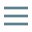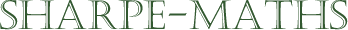Previous topic:
Introduction to expressions
Current topic:
Collecting like terms
Next topic:
Expanding expressions

Let's start by defining what we mean by the words Collecting Like Terms, which are used by many teachers and misunderstood by many students. We will start by defining what we mean by a term.

1. A term is a grouping of numbers and letters which can be combined with other terms if they are alike. Usually, terms are something like $p$ or $6x^2$ or $2pqr^3$, but we can use the word to define more complex terms which are constructed from other simpler terms. For example, $3(p-2)^2$ could be treated as a term which might be combined with other terms which also have a factor of $(p-2)^2$. So it is important to notes that some terms can have their own terms also.
2. Collecting: this means to place certain things together, according to some criteria.
3. Like: these are things which have something or some aspect or some property in common.

So collecting like terms means to take a sequence of terms and look for patterns in the terms in order to combine and simplify them.

Please look at the Examples tab for examples of how this principle works.